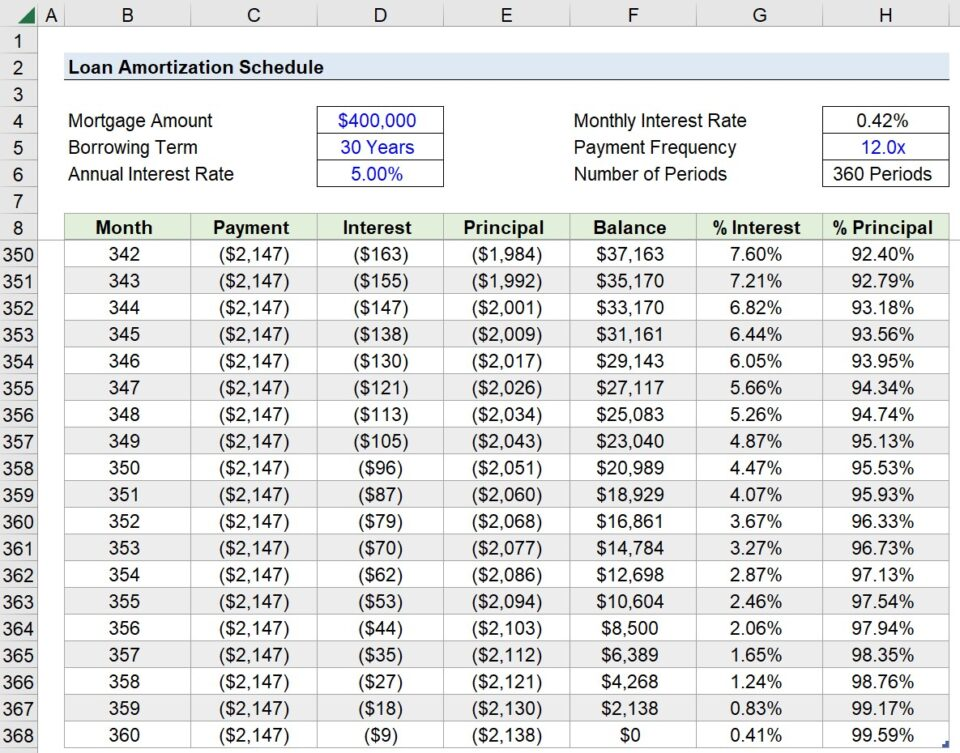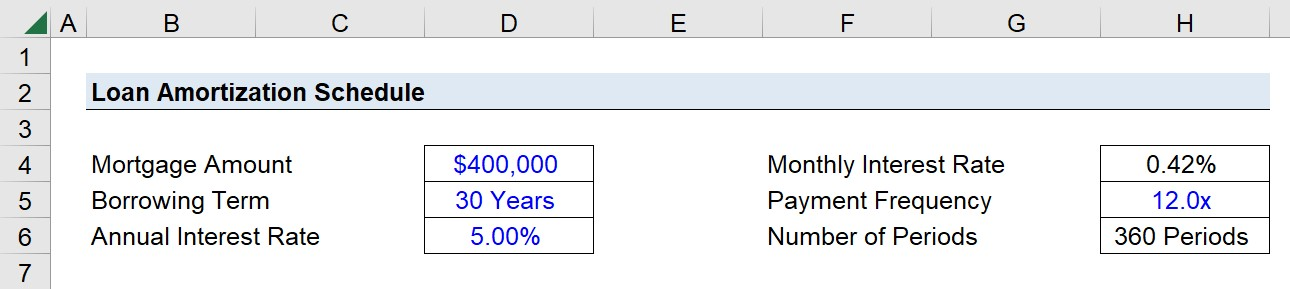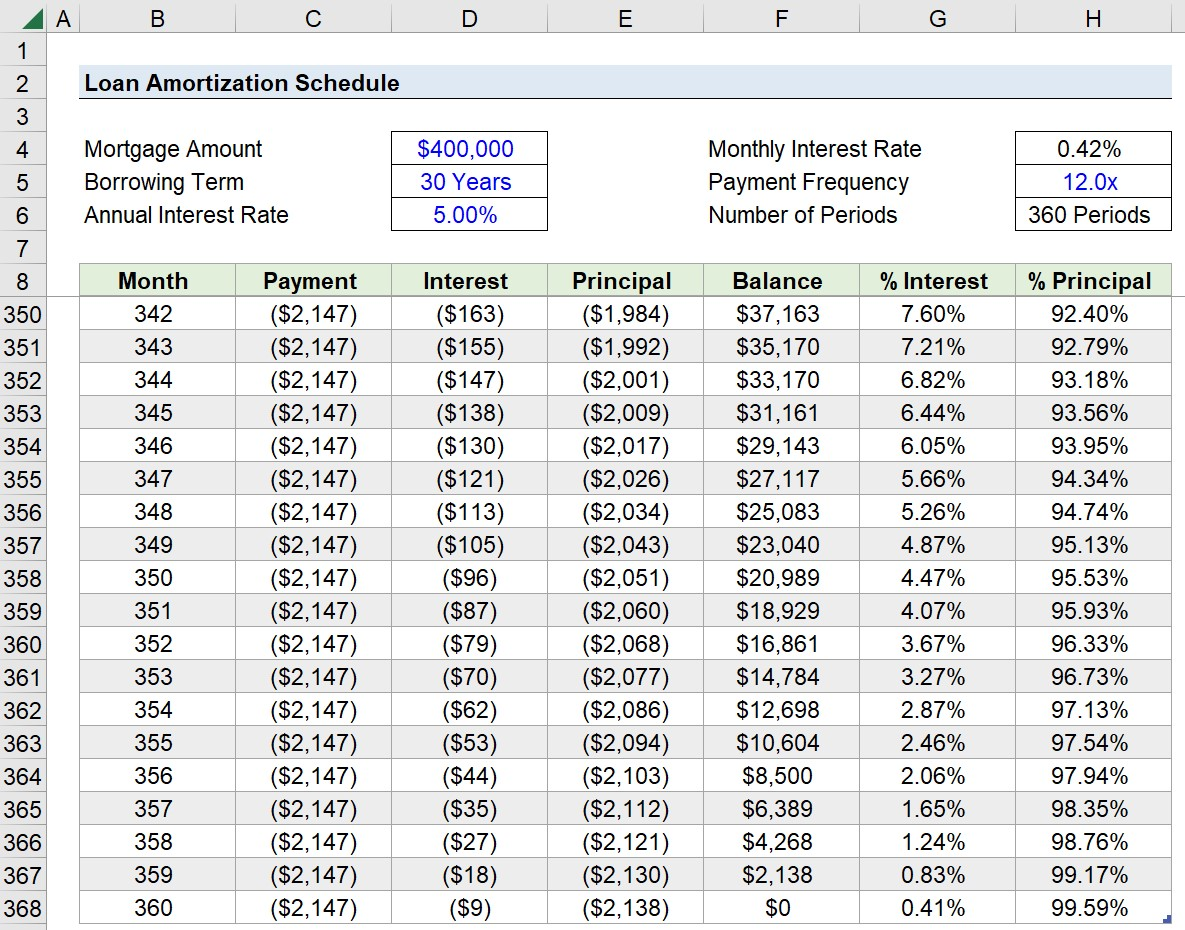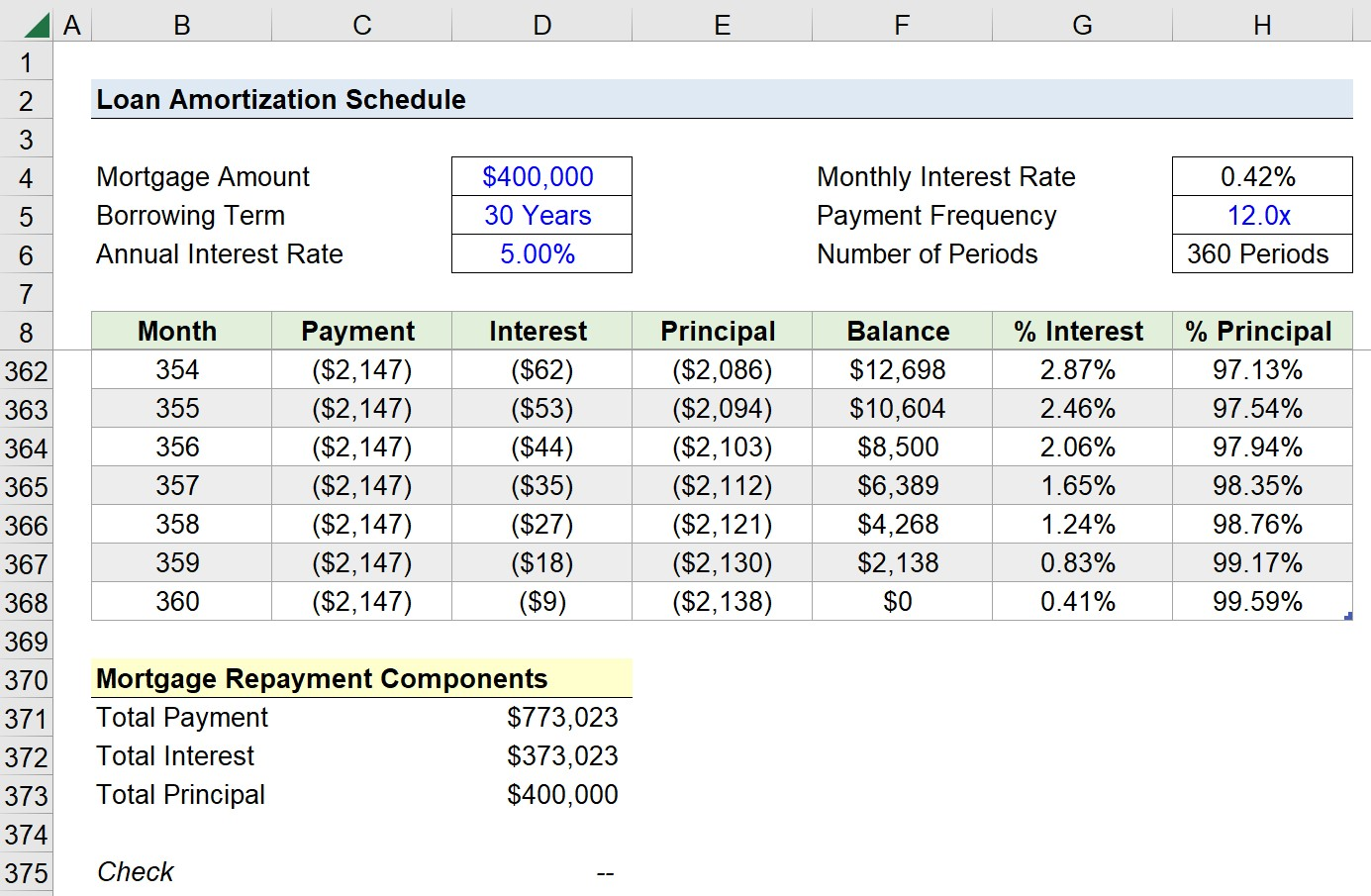Welcome to Wall Street Prep! Use code at checkout for 15% off.# Loan Amortization Schedule

Guide to Understanding the Loan Amortization Schedule in Excel## How to Calculate Amortization Schedule?

The loan amortization schedule describes the allocation of interest payments and principal repayment across the maturity of the loan.

The borrower is required to fulfill payment obligations per the schedule laid out in the contractual agreement with the lender as part of the financing arrangement.

In particular, there are two forms of payment associated with loans: 1) the interest expense and 2) the principal amortization.

• Interest Expense → The interest component reflects the cost of the borrowing, i.e. the credit risk associated with providing debt financing to the borrower is factored into the interest rate by the lender.
• Principal Amortization → The principal payments, on the other hand, represents the gradual repayment of the original principal over the maturity term.

Over the length of the borrowing term, the loan’s book value gradually reduces in value until the outstanding balance reaches zero on the date of maturity.

If the balance does in fact reach zero, that means that the borrower met all of their debt obligations on time and did not default, i.e. did not miss an interest or principal payment.## What is Fixed-Rate Amortization Schedule?

The amount of interest owed each period declines in proportion to the amount of principal repaid, despite the fixed interest rate, so the interest owed declines as more of the loan’s principal is recouped by the lender.

While the interest paid each period is in fact a function of the outstanding principal balance, interest payments do NOT reduce the principal.

Therefore, the capital at risk—the money that could be lost in the event of default—is the loan principal itself. The lender is risking losing the original loan under the belief that the borrower can meet the interest requirements and return the principal in full by maturity.

In a fixed-rate amortization schedule, which tends to be the standard among mortgage financing loans, the repayment of the loan is completed in equal installment payments.

The value of the principal and the interest payments, however, will be different between each payment period.

Starting off, a higher proportion of the total payment will go towards servicing interest. But over the course of the borrowing term, the percentage attributable to principal payments increases (and the interest payments decline).

## Loan Amortization Schedule Excel Functions

In order to create a loan amortization schedule in Excel, we can utilize the following built-in functions:

### 1. Excel PMT Function Formula

The PMT function in Excel determines the total payment owed each period—inclusive of the interest and principal payment. The total payment, unlike the other two components, will remain constant over the entire borrowing term.

=PMT(rate, nper,pv,[fv],[type])

### 2. Excel PPMT Function Formula

The PPMT function in Excel calculates the periodic principal amortization owed on the loan, which, to reiterate from earlier, should increase after each payment period.

=PPMT(rate, per, nper, pv, [fv], [type])

### 3. Excel IPMT Function Formula

The Excel PMT function calculates the interest portion of each periodic payment. Contrary to the principal payments, the interest payments should decline following each payment period.

=IPMT(rate,nper,pv,[fv],[type])

## Excel Amortization Schedule Calculator

We’ll now move on to a modeling exercise, which you can access by filling out the form below.#### Excel TemplateSubmitting...

## 1. Mortgage Loan Financing Assumptions

Suppose you’re tasked with creating a loan amortization schedule on behalf of a consumer that decided to take out a 30-year fixed-rate, fully amortizing loan.

The mortgage loan amounts to \$400,000 with an annual interest rate of 5.00% and monthly compounding.

• Mortgage Loan = \$400,000
• Borrowing Term = 30 Years
• Annual Interest Rate (%) = 5.00%
• Payment Frequency = 12x

Our first step is to convert the annual interest rate into a monthly interest rate by dividing it by 12, which leaves us with a monthly interest rate of 0.42%.

• Monthly Interest Rate (%) = 5.00% ÷ 12 = 0.42%

Since the borrowing term is denoted in years, we’ll also adjust that input to be expressed on a monthly basis by multiplying it by twelve.

• Number of Periods = 30 Years x 12 = 360 Periods (i.e. months)

The total number of compounding periods is thereby 360 periods.## 2. Amortization Schedule Calculation in Excel

With our inputs converted into the right units, we’re now ready to build our mortgage amortization table in Excel.

• Month → In the first column, we’ll enter the first month number, add one to it in the column below, and then drag the formula down until we reach our total number of periods (360).
• Payment → The next column contains the total periodic payment for each period. Hence, the amount remains fixed for the entirety of the borrowing term. Using the PMT function, we can determine that each installment payment will total \$2,147.
• Interest → The interest column is calculated using the IPMT function. As mentioned earlier, the interest should initially contribute a greater proportion in the earlier periods, but gradually reduce in value as more of the principal is paid off.
• Principal → The principal payment utilizes the PPMT function. As more payments are made, the principal amortization starts to pick up while the interest payments reduce in value. Note that only the principal amortization reduces the outstanding balance on the loan, i.e. the column to the right.
• Balance → For Month 1, we first link to our original loan principal (\$400k) and deduct the first principal payment, which is \$481. We cannot drag the formula down from here. Instead, the cell below links to the Month 1 balance (\$399.5k) and subtracts the principal payment in the matching period. After doing so, we can fill the remaining cells below and must confirm that the outstanding balance in the final period is zero.

The formula of each Excel function used in each column is as follows.

=PMT(0.42%, 360, \$400k)
=IPMT(0.42%, Month Number, 360, \$400k)
=PPMT(0.42%, Month Number, 360, \$400k)

Except for the month number, all other inputs must be an absolute reference, i.e. anchored by clicking “F4” once.

While an optional step, we’ve also added two more columns to the right (Columns “G” and “H”) to visually observe the percentage change in the interest and principal payment contribution over the course of the borrowing term.

In Month 1, the interest-principal split was 77.65% and 22.38%, but by Month 360, it shifts to 0.41% and 99.59%, as shown below.As a quick sanity check, we must confirm two items on our table to ensure there are no mistakes in our amortization schedule.

1. Interest (Column D) + Principal (Column E) = Payment (Column C)
2. Total Principal (Sum of Column E) = \$400,000

## 3. Excel Loan Amortization Schedule Analysis

In closing, we can calculate the sum of each component to determine the total mortgage repayment amounts, and we arrive at the following figures:

• Total Payment = \$773,023
• Total Interest = \$373,023
• Total Principal = \$400,00020+ Hours of Online Video Training

### Master Real Estate Financial Modeling

This program breaks down everything you need to build and interpret real estate finance models. Used at the world's leading real estate private equity firms and academic institutions.

Inline Feedbacks